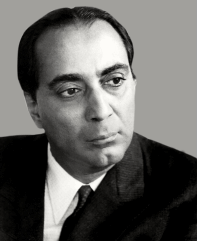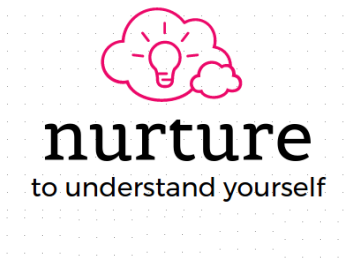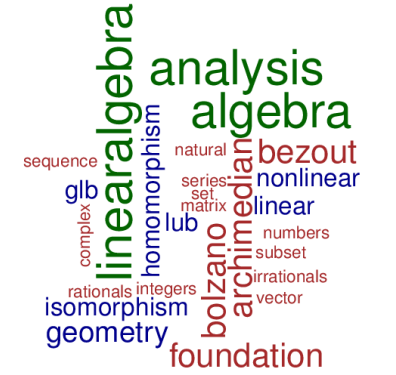nurture 2017
Winter School in Mathematics to the memory of Homi Jehangir Bhabha
4th - 23rd December 2017
10th - 22nd December 2017
Central University of Tamil NaduThis workshop will aim to touch upon some of these topics

Foundation & Algebra

• Foundation: Basic concepts of set, union, intersection, etc., lots of examples to be given. Index set, Cartesian product of sets, relations, equivalence relations, functions, 1-1 function, onto function, Partitions
• Natural numbers, Well-ordering principle, induction principle, weak induction principle and their equivalence; Divisibility, Division algorithm; GCD and LCM; Bezout’s identity. irreducible elements and prime elements; Fundamental theorem of arithmetic
• Linear Diophantine equations. Congruences; arithmetic of congruences; Chinese remainder theorem
• Composition of functions and permutations, Decomposition
• Theory of Groups, Isomorphism, Cosets and Langranges theorem, Cyclic groups, Cayley's theorem, Group action

Analysis

• Upper and lower bounds, LUB and GLB, LUB axiom
• Archimedean property, density of rationals and irrational, nested interval theorem
• Sequences in R and convergence: algebra of convergent sequences, monotone sequences, Cauchy sequences and their convergence, subsequences, Bolzano- Weierstrass theorem, recursively defined sequences
• Continuity: sequential definition, epsilon-delta definition and their equivalence. Intermediate value theorem

Linear Algebra

• Systems of linear equations, Gaussian elimination, Geometric interpretation of solution sets, non-homogeneous and homogeneous systems of linear equations. Existence of nontrivial solutions for a homogeneous system of m linear equations in n variables with m < n
• Vector spaces, vector subspaces, vector subspaces generated by a set of vectors, Linear dependence and independence, basis, dimension formula for the sum a two vector subspaces
• Linear Transformations, Kernel and image, Rank-nullity theorem, Matrix Representation w.r.t ordered bases

Workshop Poster

Homi Jehangir Bhabha
(30 Oct 1909 - 24 Jan 1966)Workshop Cloud